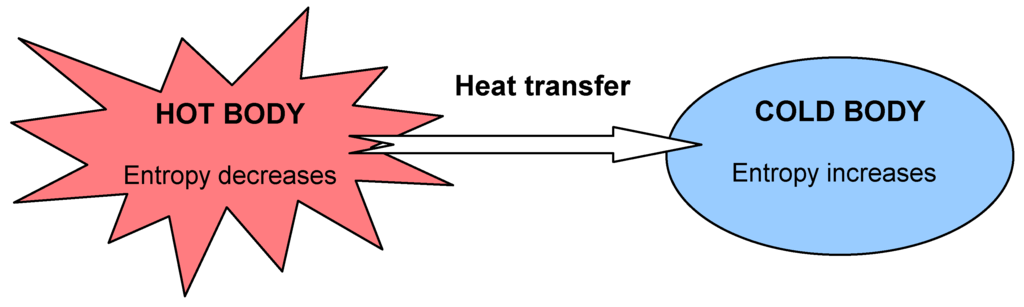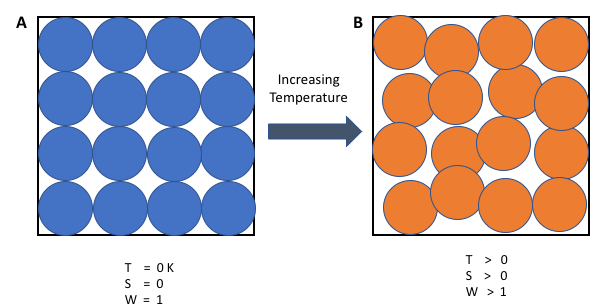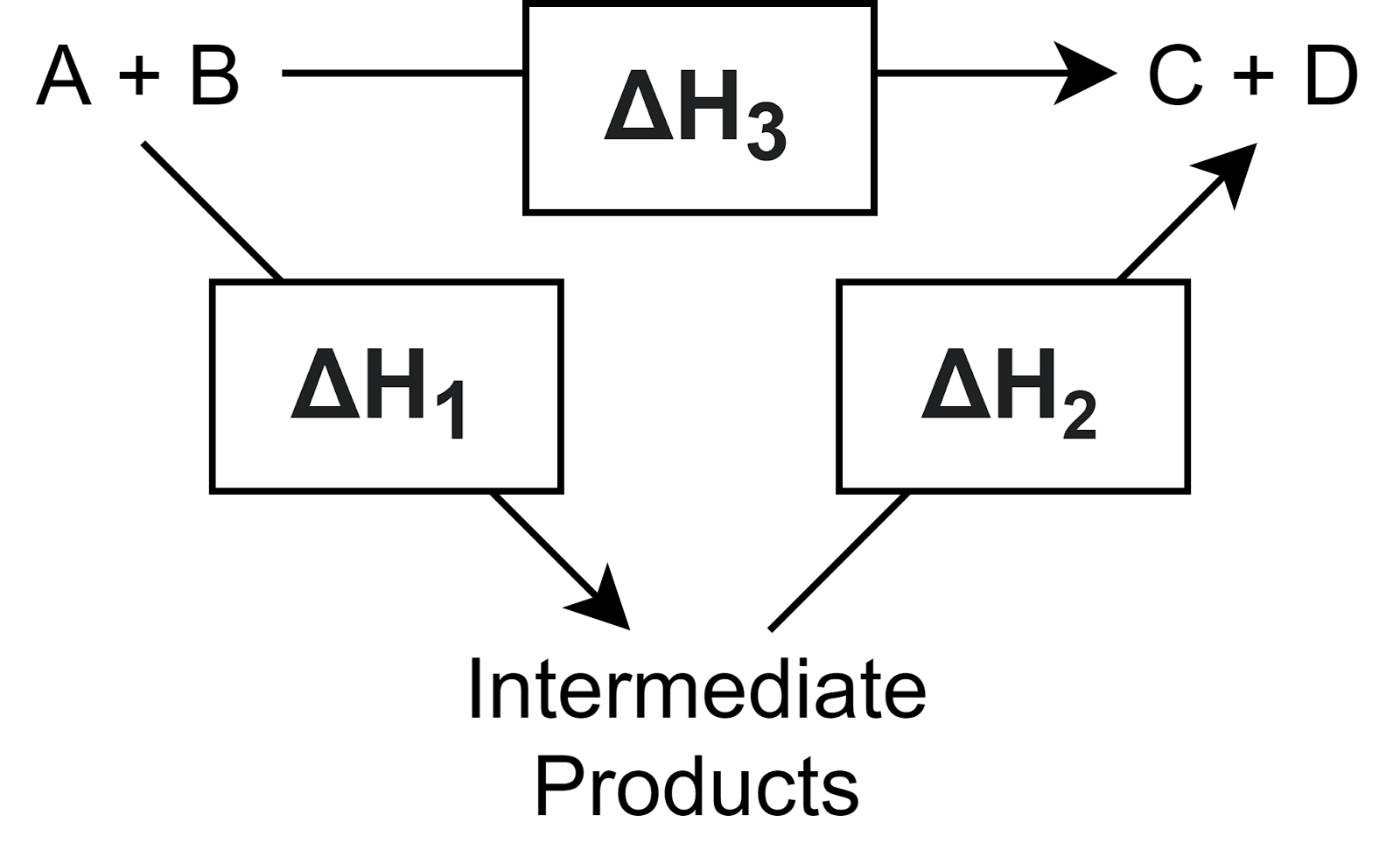Looking for revision notes that are specific to the exam board you are studying? If so, click the links below to view our condensed, easy-to-understand revision notes for each exam board, practice exam question booklets, mindmap visual aids, interactive quizzes, PowerPoint presentations and a library of past papers directly from the exam boards.

# Chemical Energetics

## Introduction to Chemical Energetics

The exchange of energy is fundamental to all chemical changes. As the exchange of energy occurs, so does the transformation in matter, and the study of these changes forms the core of chemical energetics. The roles of systems, surroundings, the flow of heat, and the laws that govern these factors are observable in complex and straightforward chemical phenomena. This article will examine how these are interconnected and what results these factors produce in unison.

## Systems & Surroundings

One of the most important aspects of chemical energetics is to define the context for studying the changes in energy exchange. The context may be defined in terms of –

System: The object under observation.

Surroundings: Everything other than the system.

Systems may get further divided into – (a) open systems where the exchange of both matter and energy is possible with the surroundings, (b) closed systems where no matter can get exchanged with the surroundings but energy can be, and (c) isolated systems which allow neither matter nor energy to get exchanged with the surroundings.

## Kinetics & Thermodynamics

A reaction can be fundamentally studied according to two viewpoints.

Kinetics – deals with the questions regarding the reaction rate and the intermediate steps involved in going from initial reactant(s) to final product(s).

### Energy and its Forms

Potential energy is the energy of a system at rest, and it depends on the place or position the system resides.

Kinetic energy – It is the energy of a system in motion, and it depends on the mass and velocity of the system.

Thermal and chemical energies – Thermal energy results from vibrating particles' kinetic energies in a system. Overall, it gets reflected as the heat. After the kinetic energy of a system is thermalized, it can only be partially converted back into potential energy, and the rest gets dispersed into the surroundings. Heat is the transfer of thermal energy between two bodies at different temperatures. As the two bodies come in contact, the collisions between the molecules result in the transfer of thermal (kinetic) energy transfer from the hotter to the colder body, and “thermal equilibrium” is attained when both substances reach an identical temperature and their molecules have the equal average kinetic energy.

Chemical energy is the potential energy stored in the chemical bonds or arrangement of molecules/atoms. During any chemical reaction, the old bonds get broken, and/or new bonds get formed. This gives rise to either emission or absorbance of heat. During this process, chemical and thermal energies get interchanged.

### Measure Energy

There exists no absolute scale to measure energy. Therefore, energy gets measured according to its ability to perform work or transfer heat. The SI unit of energy measurement is joule, and one joule is the amount of work done when a force of 1 newton acts over a distance of 1 m.

### The Spontaneity of Reactions and the Role of Heat

A spontaneous process occurs naturally under certain conditions. On the other hand, a nonspontaneous process will not take place unless it is “driven” by the continual input of energy from an external source. A spontaneous process in one direction under a particular set of conditions is nonspontaneous in the reverse direction. At room temperature and typical atmospheric pressure, for example, ice will spontaneously melt, but water will not spontaneously freeze. In many chemical reactions, heat gets supplied so that the reaction takes place, which under conditions without heat may not take place or may take a different route to produce different products.

From the perspective of a two-object system, wherein these are in contact, there is no net gain or loss of thermal energy. Instead, the available thermal energy gets redistributed among the two objects. This spontaneous process results in a more uniform dispersal of energy.## The Laws of Thermodynamics

### The 1st law of Thermodynamics

The first law of thermodynamics states that the change in internal energy of a system equals the net heat transfer into the system minus the net work done by the system.

ΔU=Q−W

ΔU is the change in internal energy U of the system. Q is the net heat transferred into the system—that is, Q is the sum of all heat transferred into and out of the system. W is the net work done by the system.

### Chemical Energy Visualized in the Form of 1st Law.

Let system A gets surrounded by B. A consists of H2 and O2. A reaction between these produces H2O. Consequently, the chemical energy contained in the erstwhile H2 and O2 finds its way out as heat which then dissipates into B. This exothermic reaction can be visualized as –

The amount of energy lost by A equals the amount of energy gained by B and therefore conforms to the 1st law of thermodynamics.

### The 2nd Law of Thermodynamics

Heat transfer occurs spontaneously from higher- to lower-temperature bodies but never spontaneously in the reverse direction.

Alternatively, it is impossible for heat transfer from a reservoir to completely convert to work in a cyclical process in which the system returns to its initial state.

In simpler terms, the second law can also be stated as “all spontaneous changes cause an increase in the universe's entropy,” wherein the term entropy means the randomness. Essentially, the transfer of energy from the system to the surroundings makes the components in the latter more energetic and hence more random.### The 3rd law of thermodynamics

The third law states the condition wherein there would be no randomness of motion of the components of a system. Hence, the entropy of a pure, perfect crystalline substance at 0 K is zero.

This is an ideal condition as the temperature of 0 Kelvin signifies no temperature and hence, no heat in the system to randomize the movement of the components.### Enthalpy – the total heat content of a system

The sum of a system’s internal energy and the mathematical product of its pressure and volume is known as enthalpy (H). It represents the total heat content of a system in its normal state. Heat itself is a measure of the flow of energy, and therefore, the enthalpy change is an important measure of how heat change affects a chemical reaction. Enthalpy change is the amount of heat released or absorbed by a system under constant pressure during a chemical or physical process. Enthalpy being a state function, does not depend on the route of the process but only on the initial and final states. A negative value of an enthalpy change, ΔH < 0, indicates an exothermic reaction; a positive value, ΔH > 0, shows an endothermic reaction. Enthalpy change also depends on the physical states of a system, and therefore, a system in its gaseous phase will have entropy different from its liquid or solid phase. This again conforms to the randomness of a system of the kinetic energy of the components of a system.

### Enthalpies of reaction – key to understanding the role of heat in reactions

Essentially, most of the reactions can be studied by considering combustion, fusion, vaporization, and solution. A system changing as a result of any of these modes invariably ends up in a state different from its initial state and, therefore, a flow of energy.

Enthalpy of combustion is the change in enthalpy that occurs during a combustion reaction, burning in oxygen. These values are usually reported as the enthalpy of combustion per mole of substance.

Enthalpy of fusion is the enthalpy change that accompanies the melting (fusion) of a substance. These values have been measured for almost all the elements and most simple compounds.

Enthalpy of vaporization is the enthalpy change that accompanies the vaporization of a substance. These values have been measured for nearly all the elements and most volatile compounds.

Enthalpy of a solution is the change in enthalpy that occurs when a specified amount of solute dissolves in a given quantity of solvent.

### Hess’s Law of Constant Heat Summation

The exchange of energy during chemical reactions can be used to find out the enthalpy of a particular step in a multiple-step reaction. Since enthalpy is a state function, this is an obvious outcome. However, the method has become popular as Hess’s law of constant heat summation. It can get stated formally as follows – The standard enthalpy of a reaction is equal to the standard enthalpies of all the steps if the reaction were to occur in multiple stages.

The important thing to note in this case is that all the steps must occur under identical temperature and pressure. The requirement for every step to be conducted in a laboratory, however, does not apply and the steps may be simply theoretical. The benefit of this process is in the theoretical estimation of the standard enthalpy of a reaction if the data for the standard enthalpy of reaction for the individual steps are known. Usually, these steps are very simple reactions involving simple molecules.## Summing up the importance of chemical energetics – explanations for energy exchange in chemical reactions

Chemical energetics is an essential field to understand and apply our day-to-day experiences with how chemical reactions involve energy exchange. The key takeaways from studying chemical energetics are –

• Heat flows from a system at high temperature to a system with low temperature till the two become equal.
• A high temperature indicates a higher random movement of constituent particles in a system and hence high entropy.
• During any chemical process, no new energy gets created. The heat that comes out in exothermic reactions is essentially the potential energy stored in chemical bonds.
• A part of the energy is often lost to the surroundings during a chemical reaction.